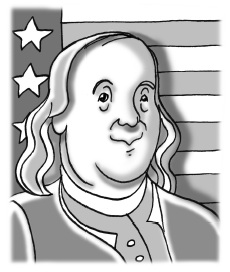### Home > PC3 > Chapter 5 > Lesson 5.2.5 > Problem5-112

5-112.

An original journal of Benjamin Franklin is up for bid at an auction house. Each bidder increases the previous bid by $25\%$. The $12^{\text{th}}$ bid is $\43,656$. What was the original bid? When will the price of the journal exceed $\1,000,000$?

$y=a(1.25)^t$

$43656=a(1.25)^{12}$
Solve for $a$.